# Bayes Theorem Summary

For the basics of Bayes Theorem, I recommend reading my short introductory book “Tell Me The Odds” It is available as a free PDF or as a Free Kindle Download, and only about 20 pages long, including a bunch of pictures.  It will give you a great understanding of how to use Bayes Theorem.

What Is Bayes Theorem – In 3 Sentences

Bayes Theorem is a way of updating probability estimates as you get new data.  You see which outcomes match your new data, discard all the other outcomes, and then scale the remaining outcomes until they are a full 100% probability.

Bayes Theorem As An Image

Medical Testing is a classic Bayes Theorem Problem.   If you know 20% of students have chickenpox, and you test every student with a test that gives 70% true positive, 30% false negative when they have chickenpox and 75% true negative, 25% false positive when they don’t.   Then before doing the test, you can construct a probability table of the 4 possible outcomes.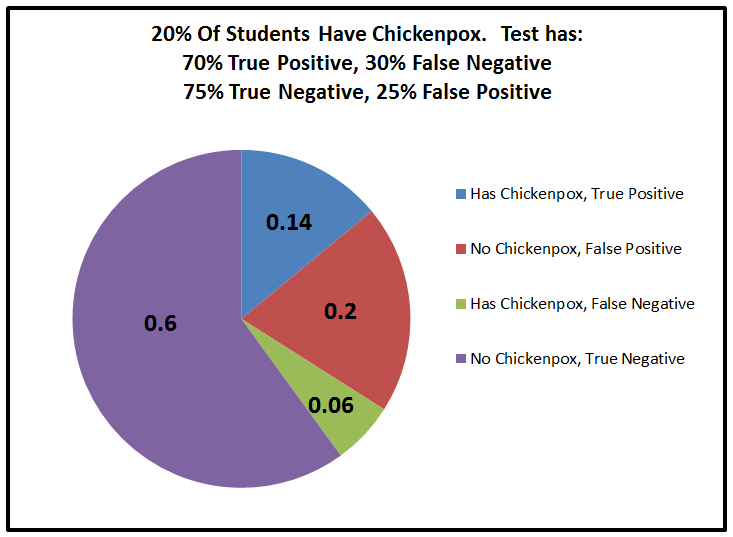This is not Bayes Theorem.  This is just a probability table.  Bayes Theorem is used when you get new data, eliminate some of the possible outcomes, and scale the other ones back up to 100% probability.  Shown belowWhen Is Bayes Theorem Used?

• The applications of Bayes Theorem are far ranging.
• It is commonly used in medical testing. For instance, you might make an initial estimate of your risk of heart disease based on the average rate of the disease in people your age, but then revise that risk once you receive new relevant information, such as your blood pressure or cholesterol results.
• Bayes Theorem was one of the first successful spam filters. You can estimate the odds that an email is spam, and then revise that estimate based on how spammy each word in the email is
• Bayes Theorem has been used to locate lost airplanes, based on what search results have turned up.

Key Points

• Bayes Theorem is just multiplication and division, with a choice of which probabilities to use
• The easiest way to think of Bayes theorem is that it is two probabilities in sequence. So list out all possible pairings of probabilities, such as in a table• And then discard any that don’t match the observed new data• And then scale the remaining probabilities until they sum to 1.0• Here is a blog post that goes into deeper detail for a medical testing problem
• Probabilities can be updated multiple times based on multiple new observations. This can be done  by doing this process multiple times in series
• One thing to be aware of is to never set any probability to zero unless you are absolutely certain it cannot occur
• This SMBC comic gives an example: Bayesian Vampire
• This XKCD comic gives an example of not taking into account the very low value of the prior, i.e. just focusing on the likelihood. Sun Exploding
• If you set a probability to zero, it will be zero forever. If you set it to a really low number, it can come back if a bunch of later observations indicate that you were in error to set it so low.
• I have a Kindle book with a number of example problems for Bayes Theorem
• A more detailed and math heavy, but very good, book is “Think Bayes”.  This is a good choice if you are familiar with Python programming

2nd Level

• Many more complicated problems start to combine Bayes Theorem with different probability distributions
• For instance, instead of assuming a uniform probability distribution for the prior, a normal distribution might be a better approximation. These refinements are problem specific depending on what probability distribution best represents the problem at hand.
• To figure out which possibilities are getting more or less probable, you can take the ratio of their likelihoods based on any given observation. This is the called “Bayes Ratio” or the “Likelihood Ratio”
• More data is a great thing. Enough data can overwhelm any approximation you made in your initial estimation of probabilities (i.e. the prior) or in your likelihood calculation.
• The easiest way to think about this is that a final probability is the product of the initial probability with all of the Bayes Adjustments from each new observation
• Prior * #1 * #2 * #3
• Eventually, the final probability is going to be dominated by all of the new observations rather than the prior

# Normal Distribution Summary

This post gives the most important points to understand for the normal distribution.  If you want to see the rest my content for statistics, please go to this table of contents

Normal Curve Basic Information

• The standard normal curve is a probability distribution, which means the total area under the curve is 1.0• Like all probability density functions (PDF), it can be done as a cumulative function, i.e. sum as you go, to show a cumulative density function (CDF)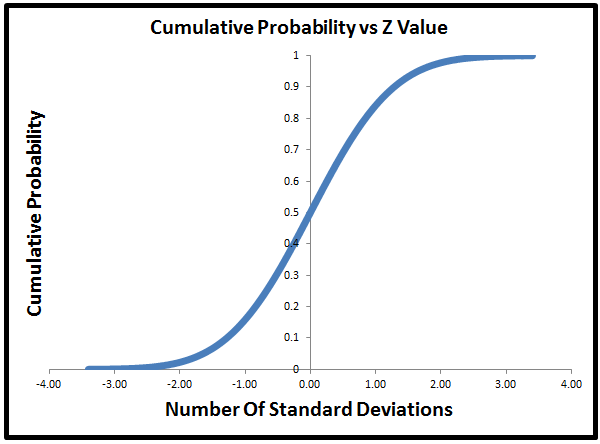• You more frequently see the normal curve plotted as a probability density function (i.e. the bell curve). But most of the time when you actually use it, such as to look up the probability of something being more than 2 standard deviations away from the mean by using a Z table, you are actually using the cumulative density function.
• To put it a different way, we more frequently care about the area under some section of the curve (the CDF at one location minus the CDF at another location) than the actual probability of any specific point on the curve.

The Real Life Meaning Of The Normal Curve

• A normal curve has a physical meaning in real life
• If you take multiple measurements of different samples, some results will end up high, some will end up low, and most will fall in the middle. The shape that results will likely be the normal curve
• The classic example of this is if you measure the height of a group of plants, i.e. how tall are different stalks of corn
• The normal curve also has an easy to duplicate mathematical meaning
• If you take a large number of independent trials of an event with 50% probability, the resulting shape will be a normal curve
• I.e. if I flip a coin 20 times and count the number of heads, and I repeat that test many times, the resulting histogram will be similar to a normal distribution
• The chart below shows the binomial distribution of 20 trials with a 50% likelihood repeated, vs the normal distribution using the same mean and standard deviation.• The more times I do that test to more similar the binomial distribution will become to the normal distribution
• This is the same reason the normal distribution exists in real life
• For instance, if we assume that a plant’s height is determined by 20 genes, and the genes the plant receives could be either tall genes or short genes, then the number of tall genes the plant gets is basically equivalent to saying how many heads will you get in 20 flips. It will follow the same distributions, which approaches the normal curve as the number of trials increases.

Using A Normal Distribution

• The normal distribution uses standard deviations as a way of fitting itself to any set of data. The standard normal distribution assumes that the standard deviation of the data set is 1.0.  You can either stretch / shrink the standard normal distribution to match your data, by multiplying it by the standard deviation, or you can shrink / stretch your data to match the standard normal distribution by dividing by the standard deviation.
• What we usually care about with a normal distribution is area under the curve up until a certain number of standard deviations
• For instance, if we want to know what percentage of the total area fall between -2 to +2 standard deviations, we would take this areaAnd we would see that 95.4% of the data falls within 2 standard deviations of the mean

• If we want to know what percentage was to the left of -1 standard deviations we would take this areaAnd see that 15.9% of the data is greater than 1 standard deviation to the left of the mean.

• Frequently this is done using a normal table, also known as a Z-table
• There are lots of good references on how to use a Z table, including this youtube video.

Level 2 Information – I recommend you get familiar with the basics of some of the other statistics topics before coming back and revisiting this in greater depth.

• Recall that the normal distribution can be generated as a series of binary events done an infinite number of times. The math of understanding binary events is the binomial distribution.  One way to approximate the binomial distribution is a normal curve. This is because the binomial distribution is just the normal curve with a finite number of events instead of an infinite number of events.
• A rule of thumb for when the normal curve is a good approximation for the binomial distribution is when the minimum of either the odds of success or failure (either p or (1-p) ) in a single trial are greater than 10. e. if I have a 50% chance of success and do 10 trials, then 0.5 * 20 = 10, so this would be a good approximation
• If I had an 80% chance of success in a single trial, and do 12 trials then I take the minimum of the odds of success (80%) or failure (20%). So this would be .2 * 12 = 2.4, so the normal curve would not be a good approximation of this binomial distribution.• The normal curve is a key component in testing for statistical significance, also known as hypothesis testing

Level 3 Information

• Not everything is a normal distribution. Some real-life events have infrequent outcomes that are more likely to occur than a normal distribution would predict.  One example of this is the financial  Occasional crashes happen with more severity than would be predicted by a normal distribution
• There are different ways of measuring a probability distribution to see how similar it is to the normal distribution
• Kurtosis is a measure of how much probability is at the center of the distribution vs at the tails
• A normal distribution has a kurtosis of 3.0, something with a fat tail would have a kurtosis greater than 3.0. This cross validated answer has a good visualization of kurtosis
• Skew is a way of measuring if the distribution is centered or not. A normal distribution is exactly symmetric.  However, a probability distribution can also be skewed left or skewed right.
• Skewed left means that the left tail is a lot longer than the right tail. This is a skewed left distribution• Skewed right (also known as positive skew, because there is more area to the right of the mean) means that the right tail has a lot more area than the left tail. This is a skewed right distribution• The normal distribution also has an equation, which is• Where µ is the mean of the data
• And σ is the standard deviation
• This is the probability distribution of the standard normal curve. Plugging in a mean, a standard deviation, and a value that you are looking for in this equation is the equivalent of using the NORM.DIST() function in Excel

Next Topic

You may want to look at Hypothesis Testing next, which heavily uses the normal distribution

# Statistical Significance Summary

This post gives the most important points to understand for statistical significance.  If you want to see the rest my content for statistics, please go to this table of contents

What Is Hypothesis Testing – In 3 Sentences

Hypothesis testing is a way of determining if some measured effect is a real difference, or likely just statistical noise.  The baseline belief is always that any difference in measurements is just statistical randomness.  We use the hypothesis testing equations to demonstrate that any measured differences are large enough that they are very unlikely to be merely random variations.

Hypothesis Testing – As An Image

Hypothesis testing is essentially placing an error band (the bell curve below) around a point that you measured (orange dot) by using a modification of the normal curve, determining where another point would be located on that chart, and seeing how much area is under the modified normal curve up until that locationThe width of the curve can change as you get more data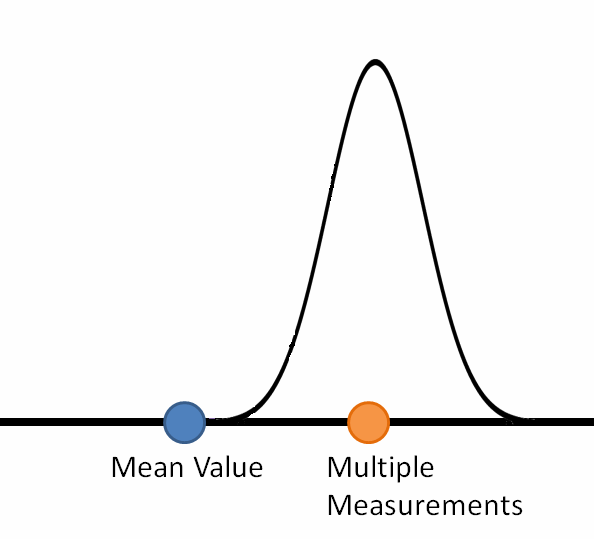And sometimes you have error bands around both pointsWhen Is Hypothesis Testing Used?

Hypothesis testing is used in scientific studies of all kinds to determine if an effect exists.  This is synonymous with the term “Statistical Significance”.   It is used, for instance, to show the difference between a real medicine and a placebo.  This is also used in things such as A/B tests for advertising to determine which ads are most effective.

Hypothesis Testing In More Detail

• Hypothesis testing always has two sets of measurements. (i.e. measure 10 samples from over here, and 15 samples from over there) Each of those two sets of measurements will have some average value.  So there are always two averages.
• Those two averages will always have some difference between them.
• Sometimes that difference is very large, i.e. if I measure the maximum weight lifting ability of a group of people before they start training vs. after they spend a year training
• Sometimes the difference is very small. Sometimes the difference can be so small it is within the precision limits of the data and shows up as zero.
• Hypothesis testing is determining if
• There is some systematic cause which results in the observed difference between the two averages or
• If it is likely that the observed difference is solely due to statistical noise, i.e. the typical fluctuations in results you get when you take measurements. This is the “Null Hypothesis”
• Example – I have a coin that I know is a fair coin and will thus come up heads 50% of the time. I flip it 100 times and get 53 heads.  The difference between 53 heads and the expected 50 heads is small enough that it is probably statistical noise rather than “Someone gave me a weighted coin”  Hypothesis testing is a way of putting concrete numbers to the statement “Probably statistical noise”
• Our default assumption is the “Null Hypothesis”. We assume that any difference in the average results is merely statistical noise until we show that to be more unlikely than a certain threshold.
• We get to decide what we want that threshold to be. A typical value is that there must be less than a 5% the results are merely random noise before we assume that the results are systematic differences.  (Less than 1% chance, and less than 0.1% chance are also common thresholds used)
• Note, even if we determine that there is a systematic difference, our calculations won’t tell us what is causing the difference in the average value between the two sets of data, just that there is at least one systematic difference
• e. if we are confident that certain groups of people are stronger after a year spent lifting weights, that doesn’t tell us if the training actually caused the difference. It could have been something different like secret steroid usage.

Hypothesis Testing Equations

• There are 5 different types of hypothesis tests, each with their own equations. However don’t get hung up on that yet, they are only small differences between all 5 of the tests.
• All hypothesis tests compare two sets of data. Call them the baseline set and the test set.
• Each of those two sets of data has 3 attributes, for a total of 6 attributes.
• The first attribute is the average of that set
• The second is the standard deviation of that set
• The third is the number of measurements in the data set
• There are 5 different types of hypothesis tests (1 Z-test, and 4 T-Tests) and the only reason there is more than 1 type of hypothesis test is that they all make different assumptions about the 6 attributes. It isn’t important to know all the different assumptions yet, but here are some examples
• One of the tests assumes that you don’t know anything about any of the 6 attributes other than what you measured
• One of the tests assumes that the only thing you know is that both sets have the same standard deviation
• Another test assumes that you have infinite measurements of the baseline set. For instance, you know the average height of people in a certain state with certainty because you looked it up in the government census results
• Other tests have different assumptions. It isn’t important to know any of these yet other than to know that the equations are doing the same thing with different assumptions
• In most cases, all 5 different types of hypothesis tests will give a similar answer. This is a good thing, and it means that if you understand how any of them work, you basically understand all of them
• This free PDF cheat sheet has the equations for the 5 different types of hypothesis tests, as well as an example of when you would use each one.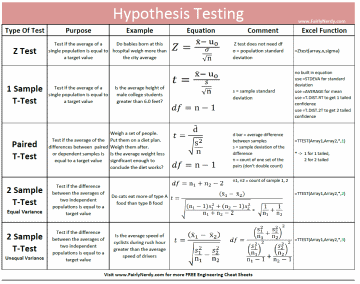Hypothesis Testing And The Normal Curve

• It is pretty important to have some knowledge of the normal curve. (i.e. bell curve), as well as a general understanding of what a standard deviation is.   See this blog post for an overview.   (blog post TBD)
• Remember that we have a baseline set of data, and a test set of data. Each of those sets has an average, a standard deviation, and a number of measurements.
• What hypothesis testing is all about is “How well do we know the average values of the populations that we took our measurements from?”
• e. even though we have an average value of our measurements, that is just the average of the samples we took, not the full population
• There will always be some difference between our sample average and the true population average
• Our average has a range of uncertainty, and we can use the normal curve and standard deviation to quantify that uncertainty
• This blog post shows how to quantify the uncertainty you have in your average value. The examples given are dice outcomes that you are already familiar with.  (i.e. that the most likely roll using 2 dice is a 7) http://www.fairlynerdy.com/intuitive-guide-statistical-significance/

T-Test Degrees Of Freedom

• Compared to the Z-test, T-tests have an additional equation, where you calculate the degrees of freedom.
• Degrees of freedom is just a way of determining how many overall measurements you have in your data set, which is used to determine how accurate your calculated standard deviation likely is.

Hypothesis Testing Examples

Level 2

That’s it for the first block of information.  If you work through a couple of examples and understand most of those points you will have a good grasp of hypothesis testing.  I recommend coming back and learning this second section in a few days or a week.

T Distribution vs Z Distribution

What is the difference between a T-Distribution and a Z distribution?

• The point of having any distribution at all is that there is a range of values that your average could be. e. having a normal distribution accounts for the fact that you don’t know your average exactly.  But you also don’t know the standard deviation of your data exactly.
• To be more precise, you know the standard deviation of your measured data. However, you don’t know the exact standard deviation of the population it was drawn from
• A Z distribution uses a normal curve and ignores any uncertainty in your standard deviation. This is because it assumes you have enough data that your standard deviation is quite accurate.
• A T distribution takes into account the fact that you have a range of error in your standard deviation. It does this by changing the shape of the distribution
• It based the shape of the curve on the degrees of freedom, which is a way of calculating how many measurements you have.
• With a T-distribution, Instead of the typical normal curve, you get a curve with fatter tails
• This applet lets you play with the shape of a T distribution vs a Z distribution assuming different number of samples http://rpsychologist.com/d3/tdist/
• To use it, slide the slider above the charts to the right or the left to change the degrees of freedom assumed in the T-Distribution
• This will change the shape of the T-distribution
• You will see that with a low number of degrees of freedom, the T distribution has much fatter tails than the normal distribution. However as the number of degrees of freedom  (i.e the number of measurements, i.e. the confidence you have in your measured standard deviation) increases the T distribution becomes nearly identical to the standard normal distribution
• Once you get around 30 data points, or so, the difference between the T Distribution and the Z distribution mostly goes away, which is why 30 degrees of freedom is a common rule of thumb on when to switch to a Z-test instead of a T-test. (Note, there other important considerations as well, such as whether you have a baseline measurement or are measuring both the baseline and the test sets of data)

When To Use Each Test

This block summarizes when you would use any given test.  As we go down this list we know less and less information and have to rely on what we measure.   I.e. instead of looking up from the government census what the average age of a region is  (i.e. knowing it), we go ask 100 people what their age is (measure it)

Z Test

• You know baseline average
• You measure sample average
• You know baseline standard deviation
• You assume sample standard deviation is the same as the baseline standard deviation

1 Sample t-test

• You know baseline average
• You measure sample average
• You don’t care about baseline standard deviation  (because we have so many baseline samples that it doesn’t matter)
• You measure sample standard deviation

2 Sample t-test – equal variance

• You measure baseline average
• You measure sample average
• You measure both sample standard deviation and baseline standard deviation but assume that they are the same as each other so you just measure them all together and do the calculations as a group

2 Sample t-test – unequal variance

• You measure baseline average
• You measure sample average
• You measure baseline standard deviation
• You separately measure sample standard deviation

The last hypothesis test is slightly different because the previous ones all assumed that what you were measuring was different groups.  The paired t-test assumes that each data point in the two sets of data are tied together.  I.e. each data point measuring the same people before and after

Paired T-test

• The average value is the average of the difference between the before and after data
• The stander deviation value is the standard deviation of the difference in before and after values

Truthfully, in many cases, you aren’t going to get very much difference no matter which of these equations you use.   Some of the equations pretty much reduce into the other equations as you get more and more information, i.e. if you measure at least 30-50 data points the difference between the 1 sample T-test and the Z test is pretty small.

Level 3 – Morphing Equations Into Each Other

It turns out that many of the equations for the 5 different hypothesis tests are just simplifications of each other.  They can be morphed into each other as you make assumptions, such as that the number of measurements goes to infinity for one of the datasets.

• Blog post on this to be done.

# How To Learn Statistics

This section of the website covers statistics and has most of the same topics that would be covered in a statistics 101 course at a University.  Here is the table of contents for the different statistics topics covered on this site.

Two good examples of free University content for this material are

Another great resource for statistics help is Cross Validated.  There you can ask questions about your specific problems and get help.

The reason I wrote this material came from my own pursuit of the same information.  I am about 10 years removed from studying aerospace engineering in college and have been working as a professional engineer since then.  However, even though I studied statistics at the university, a lot of the concepts proved to be slippery and a “use them or forget them”.  This is especially true of things I knew mostly by memorizing equations.

Remembering equations worked fine for carrying me through a semester of school, but to remember the topic for a whole career or a lifetime, it turns out that I needed something different.  I needed to find ways relate each topic other things that I knew, so it wasn’t on the periphery of my knowledge anymore, but deeply ingrained.

Benefits & Perils Of Self-Teaching

These topics are my attempt to cover statistics in a way that is easy to learn and remember long term.  A lot of this material I re-learned through self-study.  Being largely self-taught in these topics often makes it easier for me to find relatable examples for other learners than it would be for the complete expert.

The downside of self-study is that it can leave gaps.  For instance, when learning how to do multiple linear regression (i.e. draw a straight line/plane/hyperplane through data that has at least 2 independent variables) I ran across a method of doing it that was an intuitive but laborious expansion of simple linear regression.  A reader who is more of an expert than I pointed out that I had missed a simple to do (but difficult to understand) method of doing multiple regression  (i.e. Moore-Penrose pseudo inverse).

If you spot similar oversights or just plain errors, you can find my email address here, please let me know, and I can include that information for future learners

How To Learn Statistics

I think that statistics is an area where you want to go for breadth-first.  I.e. You would rather know something about a lot of topics than knowing a lot about a few topics.   My friend Kalid over at Better Explained refers to it as the Adept Method.   And a good analogy is to go for seeing a big picture first, even if it is not completely clearYou see the whole thing, but it is fuzzy

More study gradually brings it into focusmore study brings it into focus

Rather than diving deeply into specific topics before moving on.

From a practical sense, what that can mean is skimming topics on an initial read, than then revisiting the topics a couple of times as you learn other material and see the connections between them.  To facilitate this, I have set up each page with a couple different sections.  The first section of each page is designed for an initial read through to get the big picture, and the second or third sections are designed to draw connections to the other topics and to dive into a deeper understanding.  I recommend reading the first section of any given topic and then coming back a week so so later after you’ve had time to internalize some of the material, do some example problems, and look at some other topics, before diving into the second or third layer of the material

Sources Of Material

The internet has a lot of great content on it, and it doesn’t make sense to duplicate a bunch of material that already exists.  So when I know of pages that have great explanations or find useful tools, I will link to them.  And if you have suggestions please let me know.    I have also covered some of the other topics in more depth as Kindle Books (typically priced under 3 dollars), so I will link to that material where it makes sense.  I know that some people can’t access the Kindle content or are not in a position to purchase it, so I would be happy to send a free PDF copy if you contact me.

Enough Preamble

That’s all I have by way of introduction.  To get started go to

# An Intuitive Guide To Statistical Significance

## An Intuitive Guide To Statistical Significance

The purpose of this blog post is to provide an intuitive understanding of why statistical significance works the way it does.  The most important concept for that is to understand why small differences in measured results become more statistically significant as you get more measurements. And the reason for this is that the standard deviation of the mean decreases significantly as you get more measurements, even if the standard deviation of the population doesn’t change at all.

There are 5 main types of statistical significance tests.  They are

• Z Test
• 1 Sample T-Test
• Paired T-Test
• 2 Sample T-Test with Equal Variance
• 2 Sample T-Test with Unequal Variance

This blog post does not walk through all the examples and equations for each of the types.  This post gives the overarching framework that ties them all together.  If you want to see more detail about each type of T-Test you might be interested in my book  “Hypothesis Testing: A Visual Introduction To Statistical Significance

And if you want all the different equations and a quick example of when you would use each on a single page, check out this cheat sheet for hypothesis testing.  http://www.fairlynerdy.com/hypothesis-testing-cheat-sheets/This hypothesis testing summary post here a summary of some of the other key points for statistical significance not covered on this page

## What Is Statistical Significance?

When you are testing for statistical significance, the equation you are asking is, how unlikely would this outcome have been if it just happened by random chance?

You are likely aware that most real life events have some degree of scatter in the results.  If you take a bunch of measurements for similar things you will get some measurements that are high, some that are low, and some that fall in the middle.  For instance, if you are buying a prepackaged 5 lbs. bag of apples at the grocery store, and you weigh all 20 bags of apples that are on the shelf at the time, you will likely see some that are around 5 lbs., some that are 5.1 lbs, some that are 5.2 lbs, and maybe even a couple that are 4.9 lbs.

You might come in one day and measure 10 bags of apples and find that the average weight of those 10 bags is 5.2 lbs.  You might come in a couple of months later and measure 10 different bags and find that the average weight of those bags is 5.0 lbs.  Does that change in results mean that something changed to cause the new bags to be lighter?  For instance, maybe the apple packers are using more accurate machines to make sure all the weights are barely above the guaranteed 5 lbs.  Or maybe that change in results is just a random outcome of the particular bags you chose to measure on that particular day.   Statistical Significance is a way of determining which of those possibilities is true.

What we are doing with statistical significance calculations is determining how unlikely an outcome was to occur by random chance, and then deciding if that probability is unlikely enough that we can conclude something other than random chance caused that outcome.

The two most important things in a statistical significance calculation are the distance the average value of your measured data is from what you are comparing it against, and the standard deviation of what you are measuring.  It is easy to understand how the difference in measurements is important.  For instance, in the apple example, if my first group of apples weigh 7 lbs. and the second group weigh 5.0 lbs. there is more likely to be a significant difference than if my first group weigh 5.2 lbs. and my second group weigh 5.1 lbs.  The greater the difference in measurements, the greater the significance, assuming that all other values are equal.

What Is The Standard Deviation

Standard deviation is the second important topic in calculating statistical significance.  It is worth going over how the standard deviation works.  Standard deviation is a way of measuring how spread out your measured values are. If the values you are measuring are all clustered together, they will have a low standard deviation.  If they have a lot of variation in the measurements, they will have a high standard deviation.

For example, if you had measured 5 bags of apples and their weights were 5.0, 5.1, 5.1, 5.2, and 5.2 lbs, those results are a lot more tightly clustered, and hence lower standard deviation, than if you had measured 5 bags and gotten weights of 5.0, 5.6, 5.9, 6.2, 6.5 lbs.

The image below shows what we typically think of when we think about standard deviation.  There is a mean value at the center of a normal curve.   If you make another measurement of the same type of data it will fall somewhere on that normal curve, with decreasing likelihood the farther you get away from the mean value.With a typical normal curve

• 68 percent of the data will fall within 1 standard deviation of the mean
• 95 percent of the data be within 2 standard deviations
• 99.7 percent of the data is within 3 standard deviations

So if we know the mean value and standard deviation of our data, we know the distribution of results that we expect to get.  We know that if we get a result that is 2 standard deviations away from the mean, that it is a rare event because 95% of the results will fall within 2 standard deviations of the mean.

With hypothesis testing, what we are doing is turning that chart around and asking the question in reverse.  Now what we are doing is putting a normal curve around our measured data, and asking the question “How likely is it that this measured data came from the same source as the reference data?”  We are asking how many standard deviations the reference value is from our mean value.   This is shown in the chart below.Now, this might seem like it was a useless distinction.  If the reference value was two standard deviations away from the measured value, then the measured value will be two standard deviations away from the reference value.   That is completely true, but only if we only have a single piece of measured data.

## Statistical Significance Becomes Important When You Have More Than 1 Measurement

The one piece of information that you can’t figure out just using a typical normal curve is what to do with additional measurements.   Let’s say that you have a mean and standard deviation for a set of data. For instance, maybe the bags of apples on week weigh 5.2 lbs on average and have a standard deviation of 0.2 lbs.  If you go back to the store a month later and weigh a bag of apples that is 2 standard deviations heavier, at 5.6 lbs. you will think that is unusual because a 2 standard deviation difference is a rare event.  But what if your next measurement is 5.4 lbs, and your next one after that is 5.1 lbs.?  Do the additional measurements make you more or less convinced that the new bags of apples are different than the old bags?

The effect of increased quantity of measurements is the single most important concept in understanding statistical significance.  If you understand this, then you understand statistical significance. The rest of it is just knowing when to apply which equation.

The concept is this: We do not care about the standard deviation of our data. What we care about is the standard deviation of the mean value of all of our measurements. And that standard deviation of the average can change as you make additional measurements.

This is shown in the chart below. Like the chart above, it has a normal curve centered on the mean values of the measured data. However, due to the fact that there are more measurements in this data, rather than just the single measurement in the chart above, this normal curve is narrowerSince the normal curve is narrower, the reference value falls farther away from the measured average, in terms of the number of standard deviations.  As a result, we will conclude that it is less likely that our measured values and the reference value came from the same set of data.  I.e. we are less likely to get a 4 standard deviation difference than a 2 standard deviation difference unless there is an actual change that drove the difference (i.e. we are using a different group of people to pack the bags of apples this week, and they are packing them heavier.)

## Why Does The Standard Deviation Of The Mean Decrease With More Measurements?

We stated that what we are interested in is the standard deviation of the mean value of all of the measurements we make.  The standard deviation of that average value decreases as you increase the number of measurements made.  Why is that? Fortunately, we don’t have to use complicated math to explain this topic.  You have almost certainly seen it before, although you probably didn’t think about it in these terms.

The way to understand the standard deviation of the average result is to think about what happens when you roll a single die vs what happens when you roll 2 die or more dice.

If you roll a single die, you are equally likely to get a 1, 2, 3, 4, 5, or 6.  Each value will come up one-sixth of the time, so the probability distribution of a single die rolled six times will have each value coming up one time.There is no way to predict the outcome of a single die roll.   No outcome is more likely than any other.

Now, what happens if you roll two dice, and add their values? Well, there are 36 different permutations of die rolls that you can get out of two dice, 6 values from the first die, multiplied by 6 values from the second die.  However, there are only 11 different sums that you can get from those two dice, the values of 2 through 12.

Those 36 different die permutations don’t map evenly onto the 11 different possible sums. You are more likely to get values in the middle of the range than values on the edges. The probability distribution of the sum of two dice is shown below.All of a sudden, you can start to make predictions on the outcome of rolling 2 dice, even though you can’t do it for any specific die.  The single most likely sum is a 7, which is why casinos make that value a number that they win on in the game of craps.  This effect where the sum of two dice have an unequal probability distribution also shows up in the gameplay of a lot of board games, for instance, it is the key mechanic in the game “Settlers Of CatanFor our purposes, the key point is that the probability of outcomes is more concentrated in the center of the graph for two die rolls than it is for a single die roll. That concentration of probability into the center of the graph doesn’t stop with 2 dice.  If you sum the value of 3 dice there are 216 different permutations of rolls (6 * 6 * 6) mapped onto 16 possible values (3-18).   The probability distribution for 3 dice summed is shown below.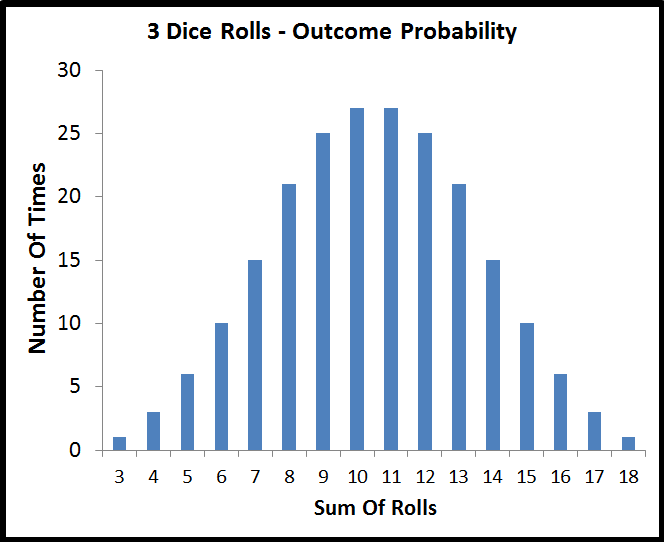Even though it isn’t quite as visually obvious as going from 1 die to 2 dice, summing 3 dice has a greater concentration of probability in the center of the graph than the sum of 2 dice. That process would continue if we kept rolling additional dice.

### The Probability Distribution Of The Average Result

So far with these dice, we’ve talked about the sum of the dice values.   Now let’s talk about the average value.  To calculate the average value, just take the sum and divide by the number of dice.  So for instance, if you rolled a sum of 7 on 2 dice, then your average value was 3.5.

The probability distribution of the average value for 1, 2, and 3 dice rolled is shown in the plot below.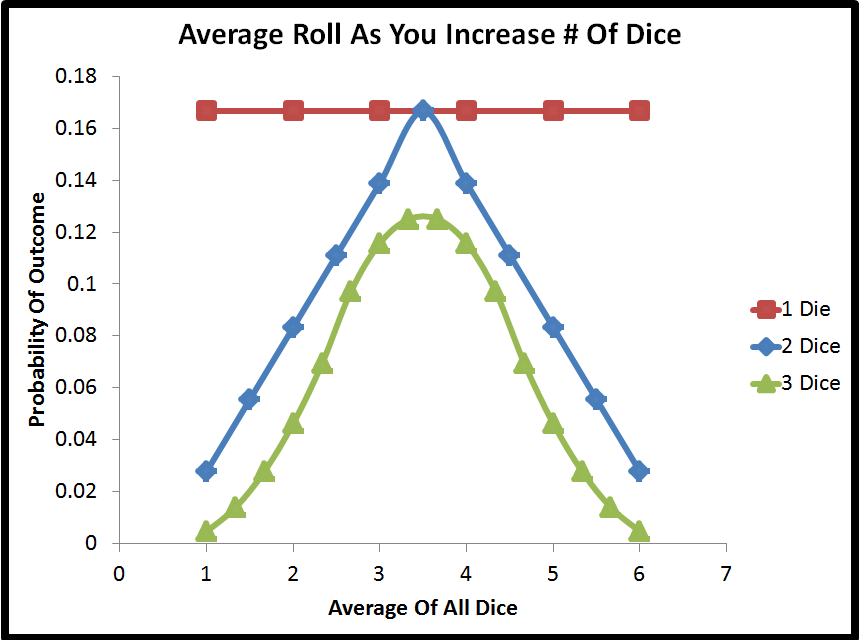This plot makes a few things obvious

• Not matter how many dice are rolled, the mean value is always centered on 3.5. This is because the average of all the numbers on a single die, (average of 1, 2, 3, 4, 5, 6) is 3.5
• As you increase the number of dice, the probability of getting an average value that is on the edges of the range decreases dramatically. The bulk of the probability shifts to the center of the graph

## Why Is This Important For Statistical Significance?

Let’s pause for a second and reiterate why we care about the above result, in terms of statistical significance.  What we see is that by making multiple measurements, we can be more confident in their average value than we can in the result of any single measurement.  The range of possible outcomes for the average of all measurements is the same as the range of possible outcomes for a single measurement, but the distribution over that range is a lot more clustered in the center.  What we are seeing is that the standard deviation of the mean of multiple measurements is lower than the standard deviation of a single measurement.   Since statistical significance is about the number of standard deviations outside of the mean value your results are, this is very important.

## Calculating The Standard Deviation Of The Average Result

In this plotIt might be surprising that the probability for every single possible average for 3 dice is lower than their counterpart for 2 dice, and also for 1 die. That is because as you increase the number of dice, the probability is spread among more possible outcomes. There are only 6 possible outcomes with 1 die, but 11 possible outcomes with 2 die and 16 possible outcomes with 3 die.   In order to get consistent probability distributions with different numbers of dice, we can use a histogram and ‘binning’ to make sure the probability is spread among the same number of outcomes.

That wouldn’t plot very well for 1, 2 and 3 dice; however here is a binned result of the probability distribution for the average roll when rolling 5, 10, and 20 dice.As you can see, the greater the number of rolls, the more the probability distribution of the average value is clustered around the average value of 3.5 and less at the edges. This means that the standard deviation of the average is decreasing as you increase the number of samples used to calculate it.

We can, in fact, calculate the standard deviation of the average for a given number of dice.  For a single die, this is just the population standard deviation of [1, 2, 3, 4, 5, 6], which is 1.7078.   For two dice, it would be the population standard deviation of the 36 possible average values (11 distinct values) of [1, 1.5, 1.5, 2, 2, 2, 2.5, 2.5, 2.5, 2.5, 3, 3, 3, 3, 3, 3.5, 3.5, 3.5, 3.5, 3.5, 3.5, 4, 4, 4, 4, 4, 4.5, 4.5, 4.5, 4.5, 5, 5, 5, 5.5, 5.5, 6], which is 1.207615.   Fortunately, there are more efficient ways to calculate the standard deviation than listing out every single value (by using a weighted average of the squared difference from the mean).  When you calculate the standard deviation of the average for all numbers of dice between 1 and 20, you get the plot below.As expected, we see that the standard deviation of the average continues to drop as we increase the number of samples.  The other notable thing about this plot is that the rate of change begins to level off.  Adding a few more dice drastically decreased the standard deviation of the average at the beginning, but it takes a greater and greater number of dice to get the same amount of change.

In practical terms, what this means for statistical significance is that there is a diminishing return to getting more data to use in your statistical significance calculation.  At the beginning additional data will make a large change, however, eventually, the cost of acquiring additional data will outweigh the benefit.

The plot below is the same as the plot above, except with a regression curve fit on the data.What we can see is that the regression fit the data exactly. The rate of change of the standard deviation of the average is equal to the standard deviation of the population, multiplied by the number of data points raised to the power of negative one-half.   A power of one-half is the same as a square root.  A negative power is the same as dividing by that number. Rewriting the equation to incorporate those changesHere the 1.7078 is the standard deviation of the population of average values with 1 sample (i.e. [1, 2, 3, 4, 5, 6]). We will denote that with a sigma. Here ‘x’ is the number of dice.   In later problems instead of ‘x’ denoting the number of dice, the equations use ‘n’ to denote the number of measurements. If we use those symbols, this equation becomesAlthough it varies slightly depending on the problem in question, that sigma and the square root of n appear in pretty much every variation of the statistical significance equations.  What they are demonstrating is that the standard deviation of the average of the samples is the standard deviation of the samples (sigma) divided by the square root of the number of samples. Or to put it another way, as you increase the number of samples, the resulting average of your measurements is increasingly likely to be close to the true population average, just like we saw with the dice rolling.

One type of statistical significance test is the Z-test.  The equation for the Z-test is shown belowWhere

• x̄ is the sample mean
• u0 is the population mean
• σ           is the population standard deviation
• n is the test sample size

As you can see, the sigma divided by the square root of n that we saw as we measured the average roll of an increasing number of dice is a key part of the equation.

# What Is R Squared And Negative R Squared

## R Squared – A Way Of Evaluating Regression

Regression is a way of fitting a function to a set of data.  For instance, maybe you have been using satellites to count the number of cars in the parking lot of a bunch of Walmart stores for the past couple of years.  You also know the quarterly sales that Walmart had during that time frame from their earnings report.  You want to find a function that relates the two, so that you can use your satellites to count the number of cars and predict Walmart’s quarterly earnings.  (In order to get an advantage in the stock market)

In order to generate that function you would use regression analysis.  But after you generated the car to profit relationship function how can you tell if it is a good quality?  After all, if you are using it to try to predict the stock market, you will be betting real money on it.  You need to know, is your model a good fit?  A bad fit?  Mediocre?  One commonly used metric for determining goodness of fit is R squared, or more formally, the coefficient of determination.

This post goes over R2, and by the end you will understand what it is and how to calculate it, but unfortunately you won’t have a good rule of thumb for what R2 value is good enough for your analysis, because it is entirely problem dependent.  Any R squared value greater than zero means that the regression analysis did better than just using a horizontal line through the mean value.  In the rare cases you get a negative r squared value, you should probably rethink your regression analysis, especially if you are forcing an intercept.

## What Is R Squared?

We will get into the equation for R2 in a little bit, but first what is R squared?   It is how much better your regression line is than a simple horizontal line through the mean of the data.  In the plot below the blue line is the data that we are trying to generate a regression to and the horizontal red line is the average of that data.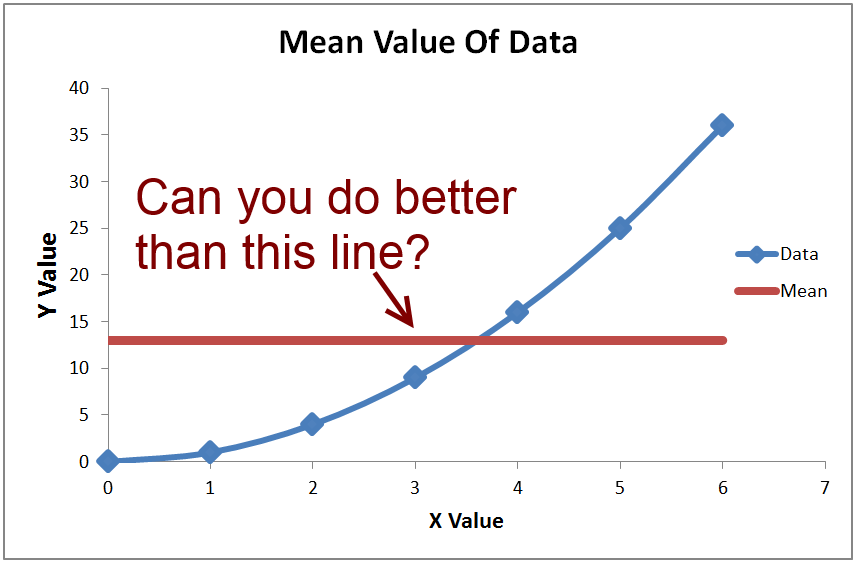The red line is the value that gives the lowest summed squared error to the blue data points, assuming you had no other information about the blue data points other than their y value.  This is shown in the plot below.  For the blue data points, only the y values are available.  You don’t know anything else about those values.   You can download the Excel file I used to generate these plots and tables here.If you want to select a value that gives you the lowest summed squared error, the value that you would select was the mean value, shown as the red triangle.  A different way to think about that assertion is this.  If I took all 7 of the y points (0, 1, 4, 9, 16, 25, 36), rearranged them into a random order, and made you guess the value of all 7 points before revealing any of their order, what strategy would give you the minimum sum squared error?   That strategy is to guess the mean value for all the points.

With regression the question is, now that you have more information (the X values in this case) can you make a better approximation than just guessing the mean value?  And the R squared value answers the question, how much better did you do?

That is actually a pretty intuitive understanding.  First calculate how much error you would have if you don’t even try to do regression, and instead just guess the mean of all the values.  That is the total error.  It could be low if all the data is clustered together, or it could be high if the data is spread out.

SS stands for summed squared error, which is how the error is calculated.  To get the total sum squared error you

• For every data point subtract that mean value from the data point value
• Square that difference

Add up all of the squares.  This results in summed squared error## Get The Regression Error

Next calculate the error in your regression values against the true values.  This is your regression error.  Ideally the regression error is very low, near zero.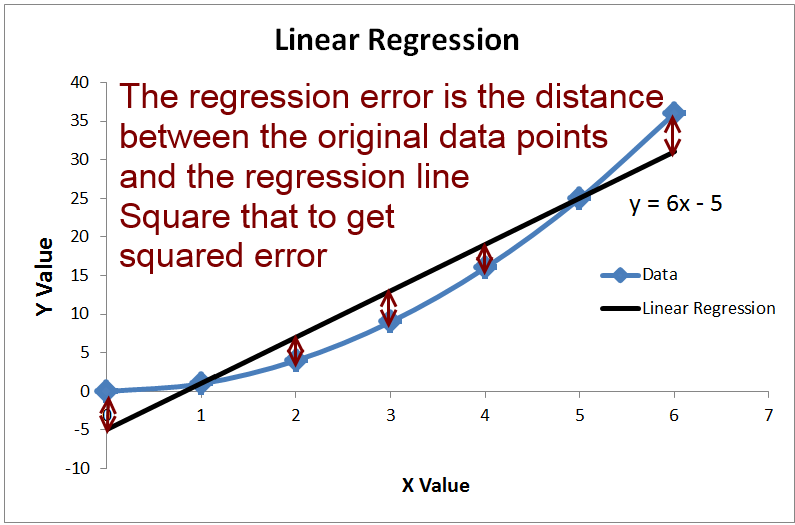The ratio of the regression error against the total error tells you how much of the total error remains in your regression model.  Subtracting that ratio from 1.0 gives how much error you removed using the regression analysis.  That is R2

In actual equation form it isTo get the total error, you subtract the mean value from each data point, and square the resultsFor the sum squared regression error, the equation is the same except you use the regression prediction instead of the mean value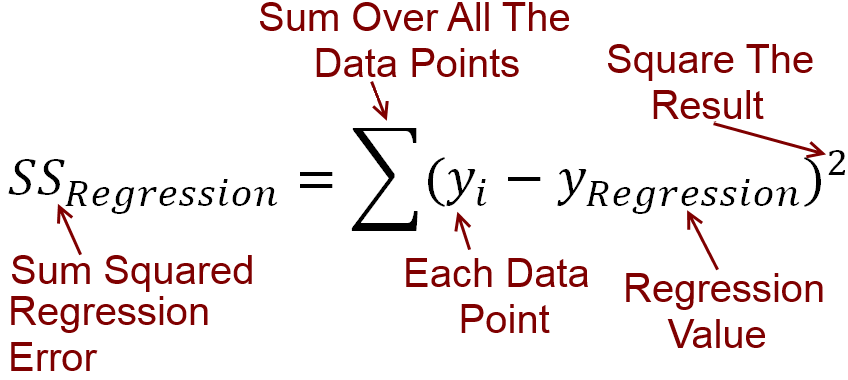## What is a Good R Squared Value?

In most statistics books, you will see that an R squared value is always between 0 and 1, and that the best value is 1.0.   That is only partially true. The lower the error in your regression analysis relative to total error, the higher the R2 value will be.  The best R2 value is 1.0.  To get that value you have to have zero error in your regression analysis.However R2 is not truly limited to a lower bound of zero.  You can get a negative r squared value.

## What Does A Negative R Squared Value Mean?

For practical purposes, the lowest R2 you can get is zero, but only because the assumption is that if your regression line is not better than using the mean, then you will just use the mean value.  However if your regression line is worse than using the mean value, the r squared value that you calculate will be negative.

For instance.  Let’s say that you wanted to make a prediction on the population of one of the states in the United States.   I am not giving you any information other than the population of all 50 states, based on the 2010 census.  I.e.  I am not telling you the name of the state you are trying to make the prediction on, you just have to guess the population (in millions) of all the states in a random order.   The best you could do here is take the mean value.  Your total squared error would be 2298.2

If you used something other than mean, for instance took the median, the summed squared error would be 2247.2

Which when converted into R2 isAnd you get a negative R2 number

## Another Way To Get A Negative R Squared Value

The most common way to end up with a negative r squared value is to force your regression line through a specific point, typically by setting the intercept.  The way the ordinary least squares regression equations work is by making a line that passes through a specified point and has the lowest possible sum squared error while still passing through the specified point.

If the point that is chosen is the mean value of x and y, the resulting line will have the lowest possible sum squared error, and the highest possible R-squared value.  If you chose the mean x and y you cannot get a negative r squared value.

However if you specify a different point for the regression line to go through, you will still get the line that generates the lowest sum squared error through that point, but that doesn’t mean that line is good.  For instance in this chart from ExcelThe intercept for both of the regression lines was set at zero.  For the regression line for the blue points, that is not too far off of the best possible regression line, and so the resulting R2 value is positive.  However for the regression line for the red points, the true intercept should be around 120, so setting the intercept to be 0 forces the regression line far away from where it should be.  The result is that the regression sum squared error is greater than if you used used the mean value, and hence a negative r squared value is the result.

The assertion that the R squared value has to be greater than or equal to zero is based on the assumption that if you get a negative R squared value, you will dump whatever regression calculation you are using and just go with the mean value.

The take away for R2 is

• An R2 of 1.0 is the best. It means you have no error in your regression.
• An R2 of 0 means your regression is no better than taking the mean value, i.e. you are not using any information from the other variables
• A Negative R2 means you are doing worse than the mean value. However maybe summed squared error isn’t the metric that matters most to you and this is OK.  (For instance, maybe you care most about mean absolute error instead)

## R Squared Example

As an example of how to calculate R squared, let’s look at this dataThis data is just the numbers 0 through 6, with the y value being the square of those numbers.  The linear regression equation for this data is

y = 6x-5

and is plotted on the graph below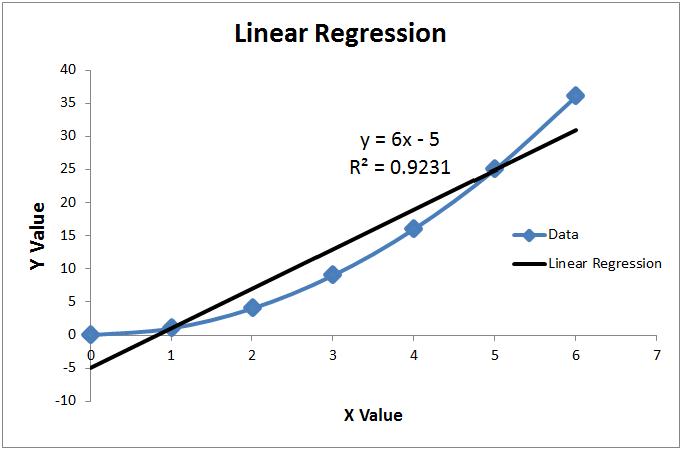Excel has calculated the R2 of this equation to be .9231.  How can we duplicate that manually?

Well the equation is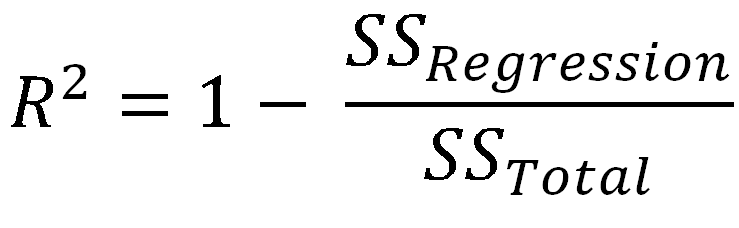So we need to find the total summed squared error (based off the mean) and the summed squared error based off the regression line.

The mean value of the y values of the data (0, 1, 4, 9, 16, 25, 36) is 13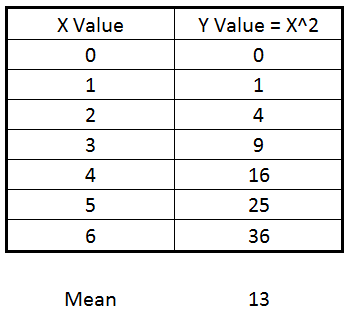To find the total summed square error, we will subtract 13 from each of the y values, square that result, and add up all of the squares.  Graphically, this is shown below.  At every data point, the distance between the red line and the blue line is squared, and then all of those squares are summed up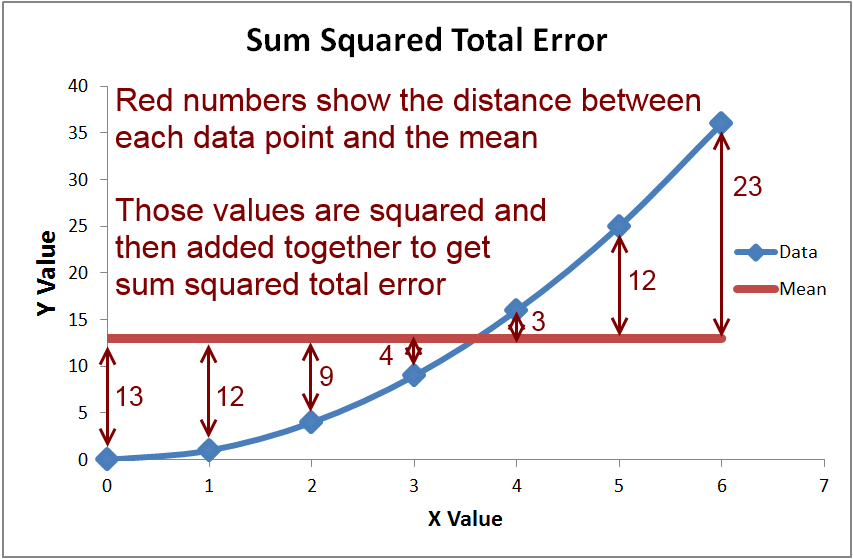The total sum squared error is 1092, with most of the error coming from the edges of the chart where the mean is the farthest way from the true value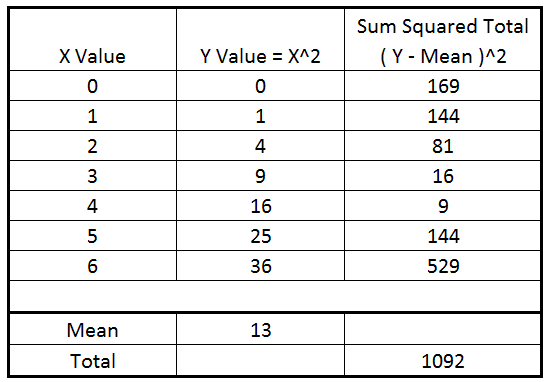Now we need find the values that our regression line of y = 6x-5 predicts, and get the summed squared error of that.  For the sum squared value, we will subtract each y regression value from the true value, take the square, and sum up all of the squaresSo the total summed squared error of the linear regression is 84, and the total summed squared error is 1092 based on the mean value.

Plugging these numbers into the R2 equation we getWhich is the same value that Excel calculated.

A different way to think about the same result would be that we have 84/1092 = 7.69 % of the total error remaining.  Basically, if someone had just given us the y values, and then told us that they were going to pick random order those y values and we had to guess what they all were, the best guess we could have made was the mean for each one.   But if now they give us the X value, and tell us to try to guess the Y value, we can use the linear regression line and remove 92.31% of the error from our guess.

But Wait, Can’t We Do Better?

We just showed a linear regression line that produced an R squared value of .9231 and said that that was the best linear fit we could make based on the summed squared error metric.  But couldn’t we do better with a different regression fit?

Well the answer is yes, of course we could.  We used a linear regression, i.e. a straight line, on this data.  However the data itself wasn’t linear.  Y is the square of x with this data.  So if we used a square regression, and in fact just used the equation y = x2, we get a much better fit, which is shown belowHere we have an R2 of 1.0, because the regression line exactly matches the data, and there is no remaining error.   However the fact that we were able to do this is somewhat beside the point for this R2 explanation.  We were able to find an exact match for this data only because it is a toy data set.  The Y values were built as the square of the X values, so it is no surprise that making a regression that utilized that fact gave a good match.

For most data sets, an exact match will not be able to be generated because the data will be noisy and not a simple equation.  For instance, an economist might be doing a study to determine what attributes of a person correlate to their adult profession and income.  Some of those attributes could be height, childhood interests, parent’s incomes, school grades, SAT scores etc.   It is unlikely that any of those will have a perfect R2 value, in fact the R squared value some of them might be quite low.  But there are times that even a low R2 value could be of interest

Any R2 value above 0.0 indicates that there could be some correlation between the variable and the result, although very low values are likely just random noise.

## An Odd Special Case For R Squared

Just for fun, what do you think the R2 of this linear regression line for this data is?Here we have a pure horizontal line, all the data is 5.0.

As it turns out, the R squared value of a linear regression on this data is undefined.   Excel will display the value as N/A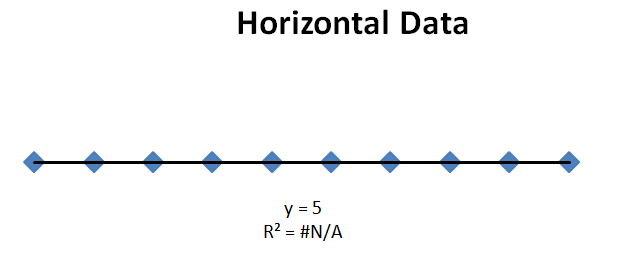What has happened in this example is that the total summed squared error is equal to zero.   All the data values exactly equal the mean value.  So there is zero error if you just estimate the mean value.

Of course, there is also zero error for the regression line.  You end up with a zero divided by zero term in the R squared equation, which is undefined.# Binomial Equation – Made Easy To Remember

The binomial equation calculates the probability of getting a certain outcome after a number of discrete events.  For instance, if your team has a 60% chance of winning any single game against another team, what are the odds that they will win at least 4 out of 7 games in the championship series against that other team?

The most common equation you see for the binomial distribution isAs far as what the letters mean

• f – just means it is a function of k, n, p
• k – This is the number of successful events. e. the total number of heads in the coin flips, or the total number of outcome A out of A/B
• n – This is the total number of events. This would be all the flips, regardless of the outcome
• p – This is a decimal number between 0 and 1 inclusive representing the probability of a successful event on a single trial. For instance if you were rolling a die and it only counted if you got a 6, then p would be 1/6 = .1667

However I think the equation is easier to remember when written in a slightly different way, asHere A is the number of times outcome A occurs, so it is equal to k from the previous equation.  B is the number of times outcome B occurs, and is equal to (n-k) when there are two events.  pa is the probability that outcome A occurs and pb is the probability that outcome B occurs

## What You Already Know In The Binomial Equation

The reason I like the equation in this form is that it builds on information that you already know.  For instance, say that you have an outcome that has a 1/6 chance of occurring, like rolling a 6 on a die.  What are the odds that event will occur 10 times in a row?   Well the odds of that happening are 1/6 raised to the 10th power.  You likely already know that, and in equation form it would beNow let’s say that you have two different outcomes, A and B.   What is the probability that outcome A will occur A times in a row, and then outcome B will occur B times in a row?   This is just the probability of A, raised to the power of A times, multiplied by the probability of B, raised to the power of B times.For instance, if you wanted to calculate the odds of rolling a die 5 times in a row and getting 6 every time, and then flipping a coin 4 times in a row and getting heads every time it would just be  1/6 raised to the 5th power, multiplied by 1/2 raised to the 4th power.  That is simple enough, easy to understand, and frankly you don’t need a special equation in order to remember it.  And that is the second half of the binomial equation.The second half of the binomial equation is just   “If outcome A had to occur all the times in a row, then outcome B all the times in a row, what is the probability that string of outcomes would occur?”

## 1st Half Of Binomial Equation

Ok, that is the second half of the equation with the exponentials, what about the first half of the equation?This is the combination equation.  There are a lot of interesting things to know about the combination equation, and those are discussed in this blog post on combinations and permutations.  For our purposes right now, the best way to think of this part of the equation is as such:   It is the number of different orders of ways you can do outcome A and outcome B.   i.e. it is the number of different tries you get at making your desired outcome happen.

As an example, let’s say that outcome A was rolling a 6 on a die, and outcome B was flipping a heads on a coin, and you needed to do that two times each.   If you had to roll a 6 twice in a row, and flip the coin twice in a row the probability would beAs we showed before.   However now let me tell you that you get to try again, however many times you can reorder the events.  For instance, the first trial was

• Roll-Roll-Flip-Flip

So that is one order.  But you can also try again

• Roll-Flip-Roll-Flip

And

• Roll-Flip-Flip-Roll

And so on.  There are in fact 6 unique orderings of 2 rolls and 2 flips.   This can be calculated by 4! / (2!*2!)  =   24 / (2*2) = 6       here the 4! Is because the total number of events is 4  (2+2)   and each of the two factorial is because there are two trials of event A, and two trials of event B.

The 6 unique orders of rolls and flips, as A’s and B’s are

• A-A-B-B
• A-B-A-B
• A-B-B-A
• B-A-B-A
• B-B-A-A
• B-A-A-B

So the first half of the equationTells you the number of times you get to try to get a specific outcome, and the second half of the equationTells you the probability of that outcome if done a single time.   Combined they give the full probability of an outcome## Example Problem Solved

Let’s take it back to the starting example.  Your team has a 60% chance of winning.  What are the odds they will win exactly 4 games in 7 ?

• Outcome A is that your team wins. This occurs 4 times, and a has a probability of .6
• Outcome B is that the other team wins. This occurs 3 times and has a probability of .4.

There are 7 events in all.  The equation becomesSo the odds of your team winning exactly 4 games are 29.03%.

## What Are The Odds Of At Least 4 Games?

But wait, the real question we asked was the odds of winning at least 4 games, not exactly 4 games.  Your team could win 5 games, or 6 games, or all 7.  That means we have to calculate the answer again for each of those possible number of wins.  That calculation is done below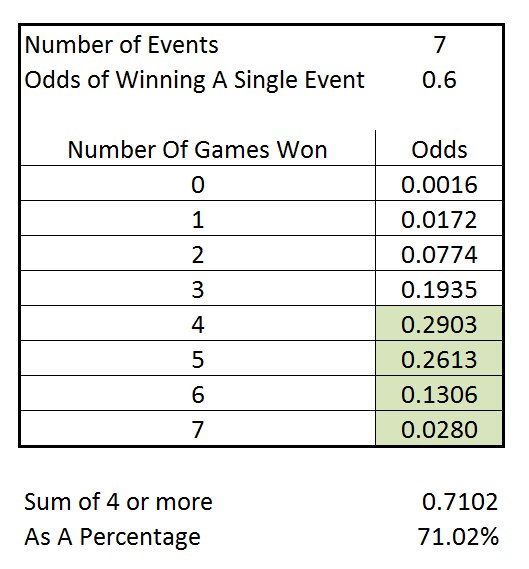The end result is that if your team has a 60% chance of winning any single game, you expect that they have a 71.02% chance of winning at least 4 games in a 7 game series.

If you increased the number of games, and went best 5 of 9, or best 6 of 11 the likelihood of the team with the higher winning percentage winning the series increases.

## Multinomial Equation

There is another reason why I like the binomial equation rewritten the way we did it.  That is because it is easy to expand that equation from a Binomial equation to a Multinomial equation.  I.e. it is easy to change from 2 outcomes to 3 or more outcomes.

What would be a multinomial event?  Well for instance, instead of having two teams play, think of three people racing in a track meet.  So instead of calculating the odds of one team winning 4 games out of 7, what are the odds that person A will win 4 races out of 7, person B will win 2 races out of 7, and person C will win one?

Here assume the odds of person A, B, and C winning any given race are 50%, 40%, and 10% respectively.

Getting the equation for 3 possible outcomes from the 2 possible outcome equation is almost trivial.  It isOnce again, the second half of the equation is the probability that that specific chain of outcomes will occur, and the first half of the equation is the number of different paths you can take to get there.

For this specific racing problem the equation becomes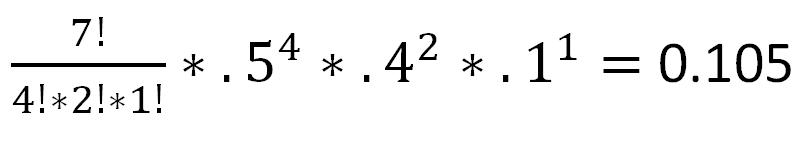Thanks for reading this post.  Please comment if there is anything not clear.   I’ve also written a longer Kindle book on this topic that has a number of more examples that you can find here.

# Dynamic Programming Time Complexity

## What Is The Time Complexity Of Dynamic Programming Problems ?

I always find dynamic programming problems interesting.  Find a way to use something that you already know to save you from having to calculate things over and over again, and you save substantial computing time.

But just how much computing time are you saving ?  One of the classic questions that you will get on any programming interview is “What is the time and space complexity of your problem?”  Which means, if you start ramping up the size of the problem with bigger numbers, will the solution get a lot slower really fast, or will it take really large numbers before the solution takes too long to be practical.

The classic way of doing dynamic programming is to use memoization.   Memoization (which looks a lot like memorization, but isn’t) means to store intermediate answers for later use.  You are increasing the amount of space that the program takes, but making the program run more quickly because you don’t have to calculate the same answer repeatedly.

## The Solution, If You Want To Skip Over The Rest Of The Post

The rest of this post goes through an example memoization program to show how it works and build an intuitive understanding of the time/space complexity.  But if you are already familiar with those type of problems and just want the answer, it is that the time and space complexity of dynamic programming problems solved using recursive memoization are nearly always equal to each other.  And they are equal to solving every sub-problem exactly one time.  So if you figure out the maximum number of sub-problems, that is your solution.

A lot of these problems can be represented on a 2 dimensional graph, so the time complexity to fill every square in the graph is N * M.  However that there are problems that are multi-dimensional (3, 4 or more dimensions), or take additional time complexity to solve a single square, so the N * M is not a generic answer.

## An Example Dynamic Programming Problem.

You have a set of coins of different denominations.  You have infinite quantities of each of the denominations.  You also have an amount of money to make change for.   How many different unique combinations of coins can you use to make exact change?

For example, if you are making change for 4 cents with coins valued 1 and 2 cents,  [1, 1, 1, 1] is unique from [1,1,2] and is unique from [2,2].  However [1,1,2] is not unique from [2,1,1].   So the solution to this small example is 3 unique ways to make change.

More importantly for this blog post the question is, when this problem is solved for an arbitrary number of coins and an arbitrary amount to make change for, what is the time and space complexity of that solution, and how can it be generalized.

Here is a larger example to see how we can solve this problem with a recursive algorithm.

For this example, assume that you have that you have 6 cents, and can make change in the denominations 1 cent, 2 cents, or 5 cents.

The recursive solution to this problem is to call the function repeatedly and each time branch into two more calls.

• On one branch subtract the largest coin from the amount remaining to make change from.
• On the other branch remove the largest coin from the list of available coins used to make change.
• If you run out of coins or get negative change, then that branch is null.
• If you end up with exactly zero money remaining, that is a solution to count.

Here is a graph of the recursive branching, without using any memorization.  (click on it for full sized image)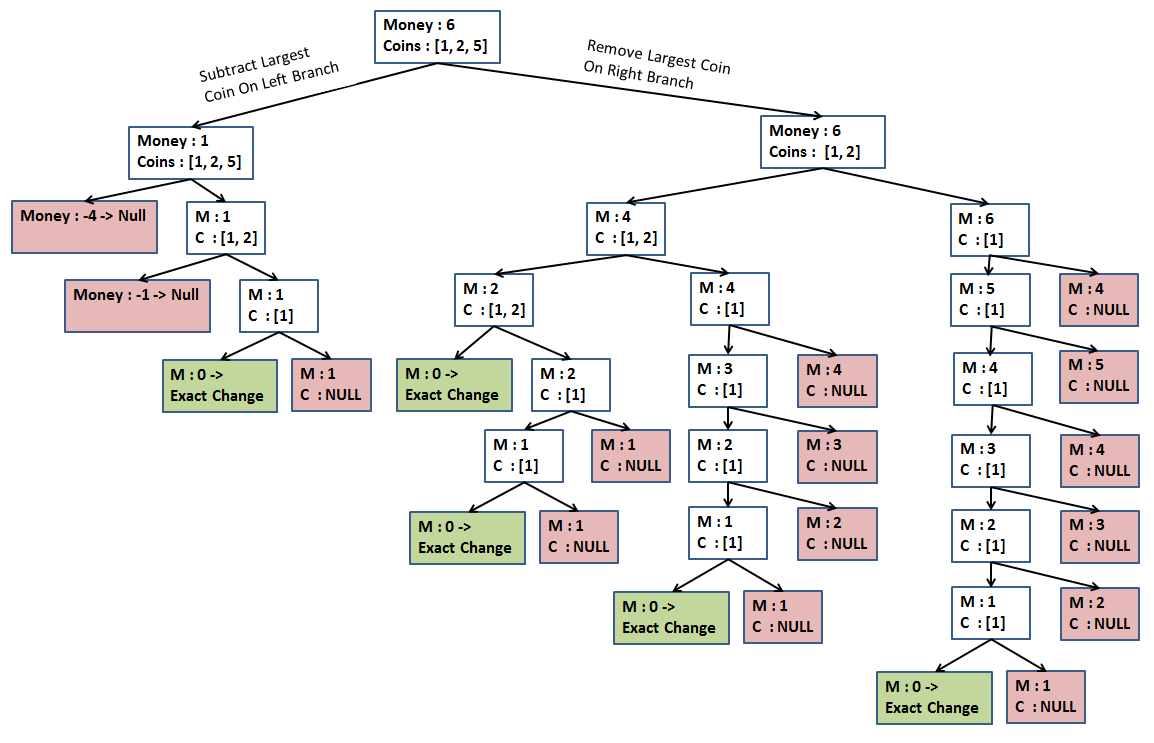That was the solution without memoization.  This was a small problem and it quickly blew up into a lot of work, as is the nature of exponential type solutions.   With memorization we get  (click for full sized image)In this chart we can see a lot fewer branches, because we re-used some of the data that we had previously computed.  Clearly the memoization saved work, since we saved re-computing several sub-problems. So how many sub-problems did we have to solve?

The answer is we had to potentially solve for every quantity of our money value (N), and every count of the number of counts we have (M).  This means that the solution will take N*M time.

And this highlights the key to the solution to the memoization time / space complexity.  For all of these type of problems you generally have to, at least potentially, solve every sub-problem, but you will only have to do it one time.  Then you can save the result to use later.

This problem had a maximum of 6 different values of change to make (1, 2, 3, 4, 5, 6) and a maximum of 3 different sets of coins [1, 2, 5], [1, 2], .   So we would have a maximum of 18 sub-problems to solve, and it would potentially take 18 blocks of memory to store that information once we solved them.  It turns out that we had slightly less than 18 problems due to the numbers that we used, but for a generalization the number of problems is on the order of N (amount of money) * M (number of different coin denominations)

The other good way to remember how much time / memory you need to solve a recursive memoization problem is to realize that the recursion is a top-down solution.  However you are solving the exact same problem as if you built the table from the bottom up.  Nearly all of these type of dynamic programming problems have a bottom up solution. ( In fact I’ve never encountered on that couldn’t be solved bottom up, but they probably do exist) Often times the bottom up solution is more difficult algorithmically, but it is easier to realize how much time and space it needs.

## If You Want To Solve This Problem Yourself

If you want to solve the coin change problem yourself, here is some sample input.  Note, these all solved nearly instantaneously on my computer with memoization in place.  without memoization, Example 3 will probably freeze your computer.

Example 1

• Amount of Money : 6
• Coin List –  1, 2, 5
• Number of possible combinations for change – 5

Example 2

• Amount of Money : 20
• Coin List –  1, 2, 4, 6, 8
• Number of possible combinations for change – 94

Example 3

• Amount of Money : 200
• Coin List –  1, 2, 4, 6, 8, 20, 35, 50
• Number of possible combinations for change – 258,822,120

## If You Want My Python Code

You can download the python code I used to solve this problem here (as a zip file).

# Kelly Criterion For Betting

## How Much Should You Bet In A Game That Favors You ?

Here is an interesting problem.  I’m going to lay out a favorable bet.  You will have the opportunity to place a bet multiple times, and resolve each one before moving onto the next one.  How much should you bet each time so that the you whose luck is exactly average will win the most money ?

Here is the gambling scenario –

• We will flip a fair coin, and if it is heads, you win.
• Before each flip you get to choose how much money you want to bet on that flip
• If you win, you double the amount of money that you bet. If you lose you lose half of the money that you bet.    e. if you bet the full 100 dollars you started with, and you win you have 200 dollars, and if you lose you have 50 dollars.
• You can play this game up to 100 times.

Now clearly this is a favorable bet.  On the first bet, if you bet 100 dollars, you have a 50/50 chance of winning vs losing.  50 percent of 200 dollars plus 50 percent of 50 dollars means the expected value of that bet is 125 dollars, for a gain of 25 dollars.

However just as clearly if you place that bet 100 times you are just as likely to win as many bets as you are to lose those bets.  So if you bet all your money each time, then times that you get lucky and win more times than you lose, for instance win 56 times and lose 44 times, you will win a lot of money.   However the person whose luck is exactly average will win 50 times and double their money, and lose 50 times and cut their money in half, and leave with the same 100 dollars they started with.

We want that average lucky person to win the most money possible, so how much should you bet ?

To simulate that I ran a python program through a couple million people betting different percentages of their bankroll. You can download that python program, the one that generated the charts below, and the associated Excel file here. This chart shows how much money the 50th percentile person would have depending on how much they bet as a percentage of their bankroll.So if you knew that you were going to win exactly 50% of your bets, your optimal bet amount exactly half of your bankroll each time.

Now if you think that you are going to be lucky, and win more than average, or you want to maximize your potential winnings you could bet more.  For a 90th percentile person, you are likely to win 56 bets out of the total 100.  That is a lot better than only winning 50 bets, but it is still a lot of losses.  This is what the winning curve looks like for the 90th percentile personEven though you were in the 90th percentile of luck, betting all your money each time would be a mistake. If you knew that you were going to win exactly 56 of your 100 bets, your optimal bet size would be 68% of your bankroll each time.  So betting more money on a positive expected value game can increase your net expected value, but it would come at an increased risk if you don’t run lucky.

## Even Winnings – In A Game That Favors You

The scenario outlined above may not be the most likely scenario you will encounter.   I have given that scenario some thought because it was in a video game that I played when I was younger.  At the end of each level you were given the opportunity to make a bet 3 times and double or halve the number of coins you had collected in the level each time.  (  Bonus points if you know what Gameboy game I’m referring to)  There are situations in business or with stock option investing that could replicate that, and you could either double your money or lose half your money, but if you are placing bets at a casino the scenario you are most likely to encounter is that you will win or lose the same amount of money.  However your odds of winning and losing may not be 50/50

Let’s say that you had a game where you would win or lose your bet, but that the odds of winning were 55% and you only had a 45% chance of losing.  i.e. imagine that you were betting on red or black on a game of roulette, except that the color distribution favored you instead of the casino. You have the opportunity place this bet 100 times.

Here betting 100% of your money is obviously a bad idea, because when you lose you will lose all of your money, and you will lose almost half the time.  The curve of winnings vs bet rate looks like this for the 50th percentile lucky personAs it turns out, the best amount to bet here is 10% of your bankroll each time.

That 10% number is suspiciously the same as the amount of advantage that you have in the game, i.e. the difference between 55% win rate and 45% loss rate.

If we run the simulation again with a 60% win rate, and a 65% win rate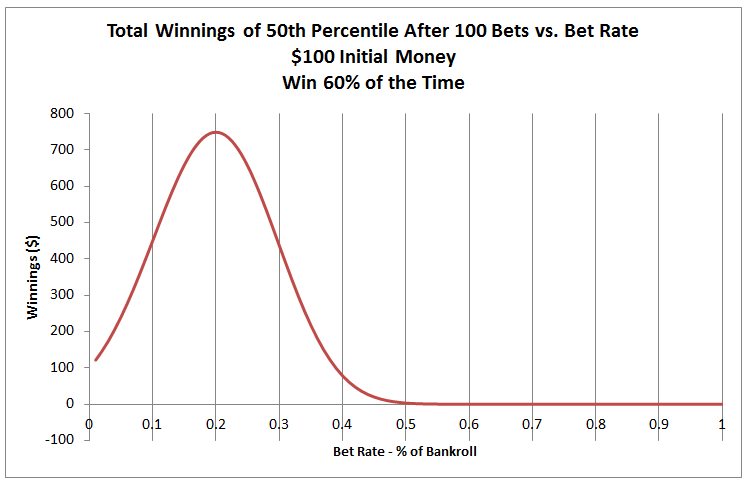We see that the best bet amount for 60% is to bet 20% of your bankroll, and the best bet amount for a 65% win rate is to bet 30% of your bankroll.    It turns out that the best bet is exactly the advantage that you have in the game.

## Kelly Criterion

It turns out that this optimal amount to bet was first discovered in 1956, and is known as the “Kelly Criterion”.   This was named after John Larry Kelly, who was an associate of the famous Claude Shannon, who went on to use this formula as well as other mathematical techniques to make a substantial amount of money in the casinos and in Wall Street.

The Kelley Criterion equation is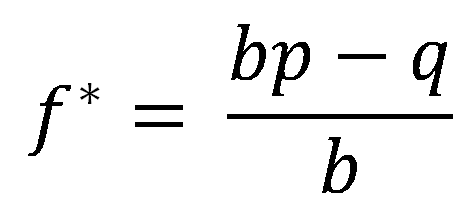Where

• f* is the percentage of the current bankroll to bet
• p is the probability of winning the bet
• q is the probability of losing the bet ( 1 – p )
• b is the net odds received on the bet. e. “b to 1”.  So for a flip of the coin, where you bet 1 and get 2 if you win (your original 1 + 1)  b would be 1.   If you are betting 1 dollar and get 6 if you win, i.e. 5 to 1 odds,  b is 5

This turns out to match our results exactly.  For the first example where we got either double or half, b is 2, and p and q are both .5.  The equation becomes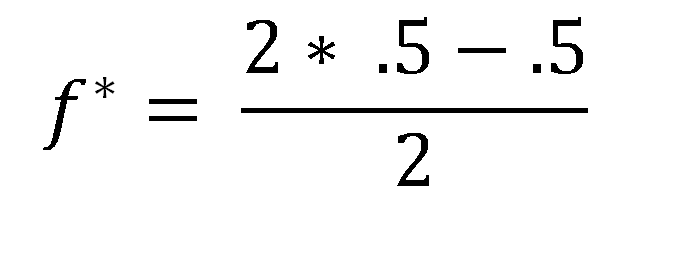And f* is calculated to be .25 = 25% of our bankroll as the optimal bet.   Now the graph indicated that we should be betting 50% of the bankroll.   However since we could only lose half of that 50% of the bankroll in the double or half bet, that is equivalent to betting 25% of the bankroll when using the same terminology as the Kelly Criterion.

For the bets where our payoff is the same as our bet, and our odds of winning are either 55%, 60%, or 65%, b is 1, and an example equation becomes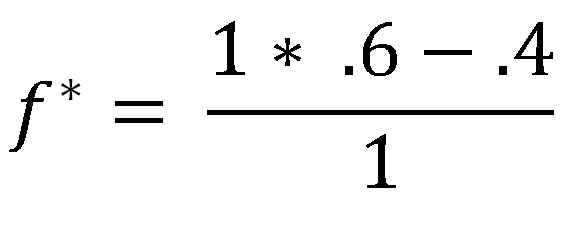Which shows that we should be betting 20% of the bankroll for the 60% chance of winning case, which is exactly the same as the python code determined.

For most real world scenarios, where you are betting against the house which has a house edge, f* becomes negative, which means that you shouldn’t be playing that game.  Truthfully it means that you should take the other side of the wager, become the house, and make them bet against you!

# Gambler’s Ruin

People have come up with many different betting systems over the years. One of these, the Martingale betting system, is interesting because it illustrates how a high level understanding of a concept can be insufficient.

## The Martingale betting system as follows:

• I will start by betting some small amount on a wager that is as close to 50/50 as I can get. So assume I’m betting \$1 on a coin flip.
• Anytime I lose, I’ll double the bet
• Anytime I win, I will start over at \$1
• Since I’m always increasing the stakes, anytime that I win it means that I will have cleared all my loses, and won an additional dollar. For instance, If I lose \$1, \$2, \$4, then win \$8 I will have lost 7 dollars, but then won 8 dollars for a net gain of 1 dollar

What the bettor appears to have is a system that guarantees them small winnings. They will steadily be winning the 1 dollar net bets.

Clearly this is not the case, from Gambler’s Ruin we know that you cannot win any negative expected value game long term. So the Martingale system is not a betting system that you should use. But why is it bad?

There is a surface issue, and an underlying math issue.

## The First Problem With The Martingale – Bet Doubling System

On the surface, one problem is that there isn’t really a game out there that is 50/50 odds you can bet on. Betting red/black in roulette is about as close as you come, but even there your odds of winning are 16/33 or 16/34 depending on the 0’s

## The Main Problem With The Martingale – Bet Doubling System

The underlying math issue is more interesting. It boils down to the fact that you cannot keep doubling your bet forever. At some point you hit either the limit of your bankroll, or the house limit. And in any finite money scenario, all you have done is set up a situation where you frequently win a little bit of money, but infrequently lose a lot of money.

So how does that work ? Let’s give an example with different amounts of bankroll.

Let’s say that you have 7 dollars total. This means that you can keep betting up to the 4 dollar limit, and if you lose the 4 dollar bet you will have lost everything. That gives you a total of 3 bets, at 1, 2, 4, before you lost.   How likely is this to happen ?

This tree illustrates the probabilitiesIf you play this game 8 times, then 7 times you will win 1 dollar, and one time you will lose 7 dollars. The net expected value from this is zero, which is exactly what you would expect since you are assuming a 50/50 game.

If you have 63 dollars, you can run through it and find that you have a 1 in 64 chance of losing the 63 dollars, and a 63 in 64 chance of winning 1 on any give series of bets.

## Your Chance Of Going Broke Is Directly Proportional To Your Bankroll

Assuming 50/50 odds, and that you have a power of 2 bankroll, your chance of going broke is exactly equivalent to your bankroll. Do you have a 1023 dollar bankroll? Then you will go broke 1 in 1024 times. Do you have a 4095 dollar bankroll? You will go broke 1 in 4096 series of bets.

What this strategy does is shift the probability curve, but the total probability remains the same. If you have a 63 dollar bankroll, and you bet 1 dollar 64 times, keeping your bet to 1 dollar regardless of if you won or lost, your probability curve would look like thisWhich can be calculated from the binomial distribution.   Doing that betting strategy, the “Normal” strategy of betting the same amount every time would give you practically zero chance of losing all your money after 64 bets.

If instead you went with the bet doubling strategy, and placed 64 strings of bets this is what the probability curve would look like.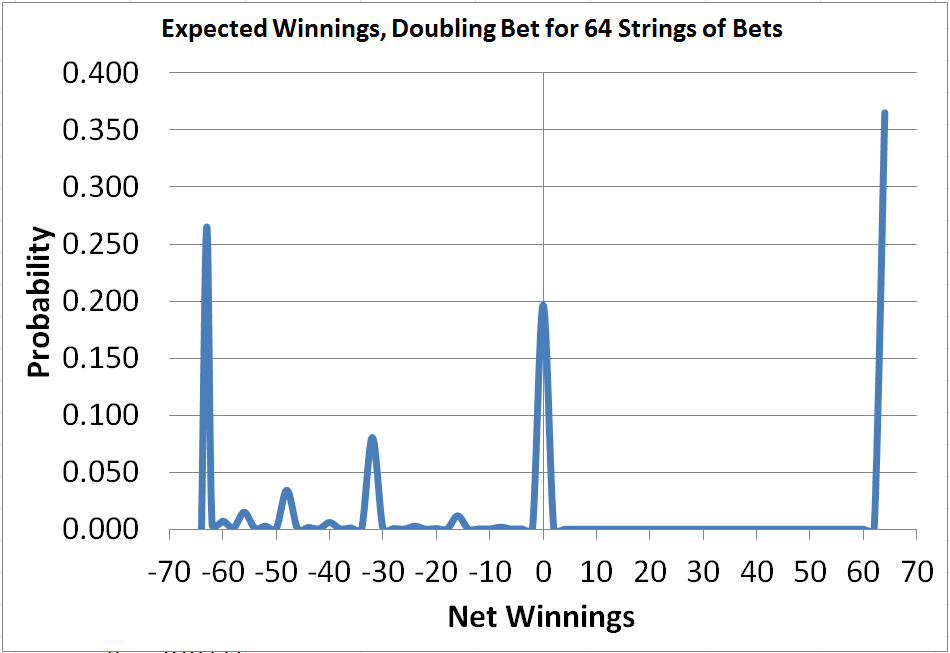I generated these odds plot with a this python program & this Excel file simulating 1 million gambling outings.   Assumptions made were

• You would place 64 strings of bets. With a string ending either when you won your bet, or couldn’t double the bet anymore
• If you lost all your money then you were done
• If you lost and couldn’t double your bet, but you still had some money you would start again at a 1 dollar bet

This chart is much different than the binomial based chart. Most of the probability is pushed to the edges of the chart, so you are most likely to either walk away having won 64 dollars, or having lost your 63 dollar bankroll.   There are other options, such as winning 30 dollars, but then losing your 64 dollar bet and ending up down ~ 34 dollars

The total expected value of that chart, which is the cumulative probability multiplied by net winnings, is zero.

So while Martingale strategy of doubling the bet strategy isn’t a surefire winning technique, it does change the outcome so that instead of being likely to win or lose a little bit, you are most likely to win or lose a lot.